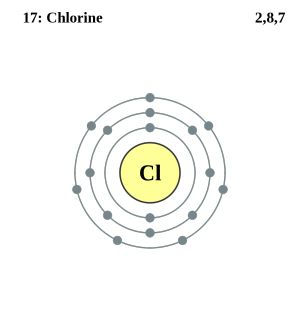# An Understanding of the Structure of an Atom – Chemistry GCSE

We all are familiar with the word atom in chemistry . It has been derived from a Greek word a-tomio which means “uncut-able” or “non- divisible”. According to the Indian and Greek philosophers the continued subdivisions of matter leads to atom which cannot be divided further. Hence they are the smallest existing particles.

## STRUCTURE OF AN ATOMImage Courtesy: livescience.com
The atom consist of three subatomic particles namely :

• PROTONS – it is the  stable subatomic particle present in nuclei of all the atoms, with a positive electric charge and equal in magnitude to that of electron .
• NEUTRONS– the subatomic particle of about the same mass as a proton, without electric charge. It is also present in the atomic nuclei except ordinary hydrogen.
• ELECTRONS-  it’s the stable subatomic particle with charge of negative electricity acting the career of electricity.

## RELATIVE CHARGE, RELATIVE MASS and POSITION OF SUBATOMIC PARTICLES IN THE ATOM

 Relative charge Relative mass Position in the atom Proton +1 1 In the nucleus Electron -1 1/2000 Revolving around the nucleus in shells Neutron 0 1 In the nucleus
• Protons have positive charge as the first letter indicates P for positive. Same is with neutrons as their first letter indicate N for neural or no charge. The left charge with us is negative so electron has negative charge on it.
• Relative masses are not the actual masses of the subatomic particles. They are the masses in relation to each other . As proton and neutron are the heaviest particles there relative masses are denoted by 1. Electrons are said to be 2000 times  lighter than both so it is denoted by 1/2000 in relation to proton and neutron.
• Now as we know proton and neutron are the heaviest particles and cannot move  so they are present in the nucleus. On the other hand electron being the lightest, revolve around the nucleus in their shells.

## ELECTRONIC CONFIGURATION

Now we know that electrons are present in the shells surrounding the nucleus, but they cannot be arranged randomly. They occupy the shells according to their electronic configuration which is the distribution of electrons of an atom or molecule in the orbitals in a specific pattern.
Electron holding capacity of the shells

 Shell 1 2 electrons Shell 2 8 electrons Shell 3 8 electrons Shell 4 16 electrons
EXAMPLE
Sodium (Na) has 11 electrons so the electronic configuration will be – 2,8,1Image courtesy: Pinterest

Chlorine (Cl) has 17 electrons so the electronic configuration will be – 2,8,7Image courtesy: wikimedia

## REPRESENTATION OF ATOM IN PERIODIC TABLEImage courtesy: olevelchemistry.com
• The symbol of respective elements are written at the place of X in the picture above.
• A represents the Atomic mass / mass number of the element which is equal to the number of nucleons ( proton and neutron) in the nucleus .
• Z represents the Atomic number which is equal to the number of protons in a nucleus ( also remember the number of protons is equal to the number of electrons )
We can summarise this concept with following formulas:
Atomic number (Z) = the number of protons present in the nucleus
Mass number (A) = number of protons + number of neutrons
Number of electrons = Z
Number of neutrons = A-Z
Example :  6C12
With this example we can find out the values of all the three subatomic particles :
Here the Atomic number (Z) is 6 = the number of protons = the number of electrons
Number of protons = 6
Number of electrons = 6
The mass number (A)= 12
A = number of protons + number of neutrons
12= 6 + number of neutrons
Number of neutrons = 12-6 = 6
ISOTOPES
Isotopes are the atoms with same number of protons but different number of neutrons. Since the Atomic number is equal to the number of protons and mass number is the summation of protons and neutrons, we can also define isotopes are the element having same Atomic number but different mass number.

For example :
6C12            6C13           6C14
We have 3 isotopes of carbon having same Atomic number but different mass number. On the basis of calculations taught in the previous section we can take out the values of subatomic particles present in all three of them to satisfy our first definition of isotopes.

 6C12 6C13 6C14 Protons 6 6 6 Electrons 6 6 6 Neutrons 6 7 8

Mahima Laroyia, is an Oxford Postgraduate in Integrative Biosciences who has more than 8 years of tutoring experience in tutoring students Maths and Sciences from KS2 up to A-levels. She specializes in providing custom and tailor made study materials to school students to make Maths and Sciences easier and fun.Find me at: www.expertguidance.co.uk